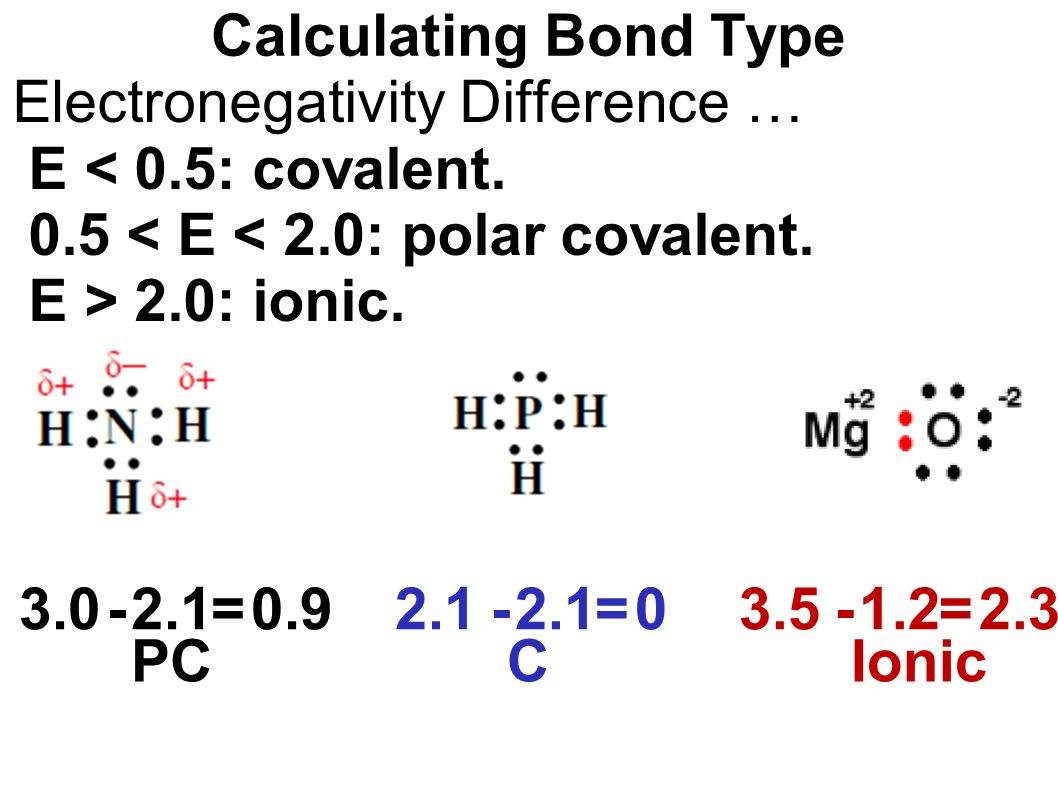# How to Calculate Electronegativity of Element

Being the student of basic chemistry in our elementary classes most of us often calculate electronegativity, as it is the fundamental concept of chemistry.

In the chemistry branch of science electronegativity in simple language can be defined as the concept, in which we consider the tendency of an atom to attract electrons towards it. Further, this tendency of an atom to attract other electrons is based on several factors, such as the allotted atomic numbers to that atom and also the distance of atom’s valence electrons from the nucleus.The intensity of atom’s tendency to attract electrons can be figured by its number and the higher this number is more will be its tendency to attract electrons, which is also known as the electronegativity number of atom.

For example, if we analyze two chemical elements Chlorine and hydrogen for their electronegativity. Then we will find that chlorine has more electronegativity than hydrogen element.

This is the reason why chlorine would be having closer bonding electrons in the comparison of hydrogen.

In a similar manner, you can easily check out the tendency of an atom for this electronegativity process by simply figuring out the electronegativity number of such items.

There are many other aspects that contribute to the calculation of electronegativities. Such as the nuclear charges and the presence of atomic shells and it is a broad concept in itself. That is studied at an intermediate or advanced stage of chemistry.

## How to Calculate Electronegativity?

If you have built up the basic understanding of electronegativity. Then it’s time to move on to the calculation of figuring out electronegativity for any atom. This is something which you will be often asked in your examination to be solved or even for your basic knowledge of chemistry.

Here below is our step by step guide by which you can calculate electronegativity of any desired atom.

Kindly note- we are using the Mulliken electronegativity formula here to figure electronegativity among several other formulas.

• At the very first step, you have to calculate the ionization energy of the atom. For which electronegativity has to be calculated.
• You can refer to chemistry materials to find this ionization energy, For example, the ionization energy of lithium is 520.
• Now as a second step figure out the affinity of the same atom. As it is also required in the process.
• Now you simply need to solve this equation in order to find out the electronegativity of an atom.

So, this is quite simple to calculate the electronegativity of an atom by using this Mulliken formula. You can take any chemical’s atom here to check its electronegativity.